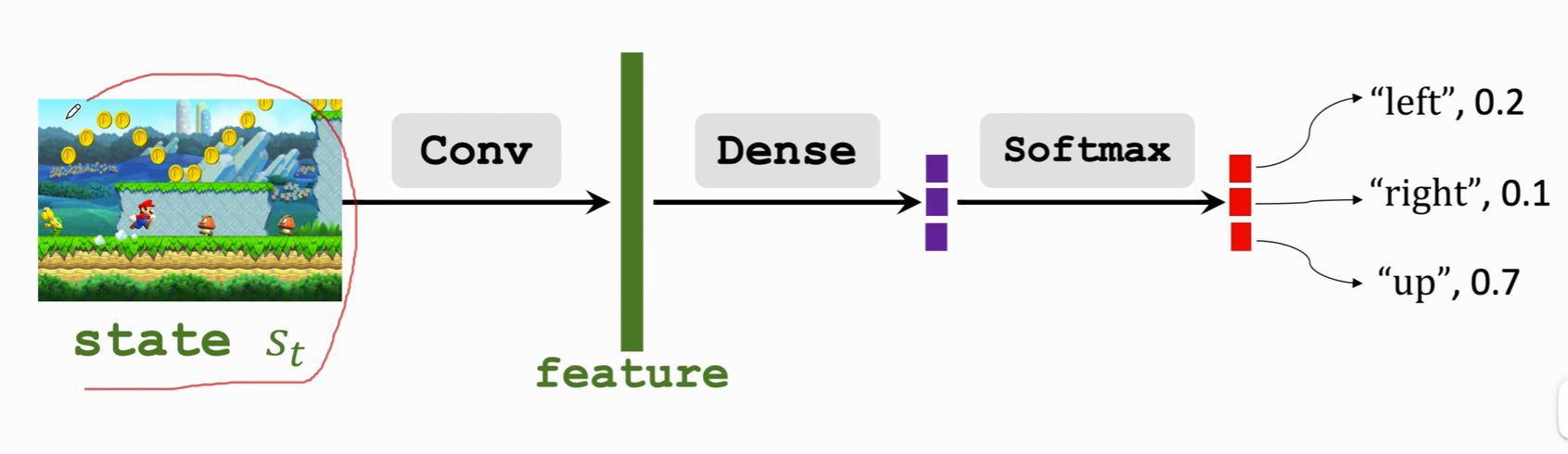# 强化学习-学习笔记3 | 策略学习

Policy-Based Reinforcement Learning. 策略学习。

## 3. 策略学习

#### 3.2 策略网络

Policy Network.#### 3.3 状态价值函数回顾

State-Value-Function.

• $$U_t=R_t+\gamma R_{t+1}+\gamma^2 R_{t+2}+\gamma^3 R_{t+3}+\cdots$$

• $$Q_\pi(s_t,a_t) = \mathbb{E}[U_t|S_t=s_t,A_t=a_t]$$
• 评价在状态 $$s_t$$ 的情况下做出动作 $$a_t$$ 的好坏程度。

• $$V_{\pi}(s_t) = \mathbb{E}_A[Q_\pi(s_t,A)]$$
• 消掉了动作 A ，这样 $$V_\pi$$ 只跟状态 s 与策略函数 $$\pi$$ 有关了。
• 给定 $$\pi$$，可以评价当前状态的好坏；给定状态$$s_t$$，可以评价策略 $$\pi$$ 的好坏。
• 展开：
• $$V_{\pi}(s_t) = \mathbb{E}_A[Q_\pi(s_t,A)]=\sum_a\pi(a|s_t)\cdot Q_\pi(s_t,a)$$这里动作是离散的。
• $$V_{\pi}(s_t) = \mathbb{E}_A[Q_\pi(s_t,A)]=\int\pi(a|s_t)\cdot Q_\pi(s_t,a) da$$这里动作是连续的

#### 3.4 策略学习的主要思想

$V_{\pi}(s_t) = \mathbb{E}_A[Q_\pi(s_t,A)]=\sum_a\pi(a|s_t)\cdot Q_\pi(s_t,a)$

• 用策略网络 $$\pi(a|s;\theta)$$ 来近似 $$\pi(a|s)$$

• $$\pi(a|s_t)$$ 函数替换成 $$\pi(a|s_t;\theta)$$，即为：

$$V(s_t;\theta) = \sum_a\pi(a|s_t;\theta)\cdot Q_\pi(s_t,a)$$

J 函数可以理解为，使用策略函数 $$\pi$$ ，agent的胜算有多大。

• 观测到状态 s，这个 s 是从状态的概率分布中随机抽样出来的。

• 把$V(s;\theta)$关于 s 求导可以得到一个梯度，然后用梯度上升来更新 $$\theta$$$$\beta$$是学习率。

$$\theta \leftarrow \theta +\beta\cdot \frac{\partial V(s;\theta)}{\partial \theta}$$

#### 3.5 策略梯度算法

##### a. 两种形式
1. $$\pi$$ 关于 θ 的导数 ✖ $$Q_\pi$$，再做连加；

$$\frac{\partial V(s;\theta)}{\partial \theta}=\sum_a\frac{ \partial \pi(a|s;\theta) }{\partial \theta}\cdot Q_\pi(s,a)$$

2. $$\log\pi$$ 关于 $$\theta$$ 的导数，乘以 $$Q_\pi$$，再关于随机变量 A 求期望。

$$\frac{\partial V(s;\theta)}{\partial \theta}=\mathbb{E}_{A\sim\pi(\cdot|s;\theta)}[\frac{ \partial log\pi(A|s;\theta) }{\partial \theta})\cdot Q_\pi(s,a)$$

##### b. 计算梯度

$$\frac{\partial V(s;\theta)}{\partial \theta}=\sum_a\frac{ \partial \pi(a|s;\theta) }{\partial \theta}\cdot Q_\pi(s,a)$$

1. 对于每个动作 a , 计算 $$f(a,\theta)=\frac{\partial{\pi(a|s;\theta)}}{\partial\theta}\cdot{Q_\pi(s,a)}$$

2. 策略梯度就是把 每个动作的 f 值 加起来：

$\frac{\partial{V(s;\theta)}}{\partial \theta}=f(a_1,\theta)+f(a_2,\theta)+...+f(a_n,\theta)$

$$\frac{\partial V(s;\theta)}{\partial \theta}=\mathbb{E}_{A\sim\pi(\cdot|s;\theta)}[\frac{ \partial log\pi(A|s;\theta) }{\partial \theta})\cdot Q_\pi(s,a)$$

1. 根据概率密度函数 $$\pi$$ 随机抽样得到一个动作 $$\widehat{a}$$ ,

2. 计算 $$g(\widehat{a},\theta)=\frac{\partial{log\pi(\widehat{a}|s;\theta)}}{\partial\theta}\cdot Q_\pi(s,\widehat{a})$$

注意这里的 $$\widehat{a}$$是抽样出来的已确定的值。

3. 根据公式2，g 函数 关于 A 求期望即为策略梯度:

$$\mathbb{E}_{A}[g(\widehat{a},\theta)] = \frac{\partial V(s;\theta)}{\partial \theta}$$

4. 由于 $$\widehat{a}$$ 是随机抽出来的，，所以 g 函数是 策略梯度的无偏估计。

5. 由于4中结论，所以可以使用 g函数来近似 策略梯度，这就是蒙特卡洛近似。

mark 一个课程 CS285 Lecture

##### c. 算法过程
1. 在 t 时刻观测到状态 $$s_t$$ ，接下来用蒙特卡洛近似来计算策略梯度
2. 把策略网络 $$\pi(\cdot|{s};\theta)$$ 作为概率密度函数随机采样动作 $$a_t$$
3. 计算价值函数的值，记作$$q_t \approx Q_\pi(s_t,a_t)$$
4. 对策略网络 $$\pi$$ 求导，得到向量矩阵或者张量：$$d_{\theta,t}=\frac{\partial log \pi(a_t|s_t,\theta)}{\partial \theta}|\theta=\theta_t$$
5. 近似计算策略梯度：$$g(a_t,\theta_t) = q_t \cdot d_{\theta,t}$$
6. 更新策略网络：$$\theta_{t+1}=\theta_t+\beta \cdot g(a_t,\theta_t)$$，梯度上升，为了让价值函数 V 变大。

$s_1,a_1,r_1,s_2,a_2,r_2,\cdots,s_t,a_t,r_t$

## x. 参考教程

posted @ 2022-07-05 11:55  climerecho  阅读(593)  评论(0编辑  收藏  举报